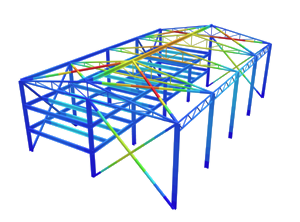SkyCiv Documentation

Your guide to SkyCiv software - tutorials, how-to guides and technical articles

1. Home
2. SkyCiv Structural 3D
3. Members
4. Curved and Spiral Members

# Curved Members

SkyCiv provides a number of advanced operations to speed up your modeling workflow.

The ‘Curved member’ operation allows you to select (2) nodes and generate an arc (made up of members) between them. This feature is useful when modeling curved structures like curved beams or arches.

## Example: Modelling a curved beam

In this example we will model a curved beam. The first step is to create two nodes. Use coordinates (0,0,0) and (10,0,0) meaning that our two nodes are separated by 10 ft in the X direction.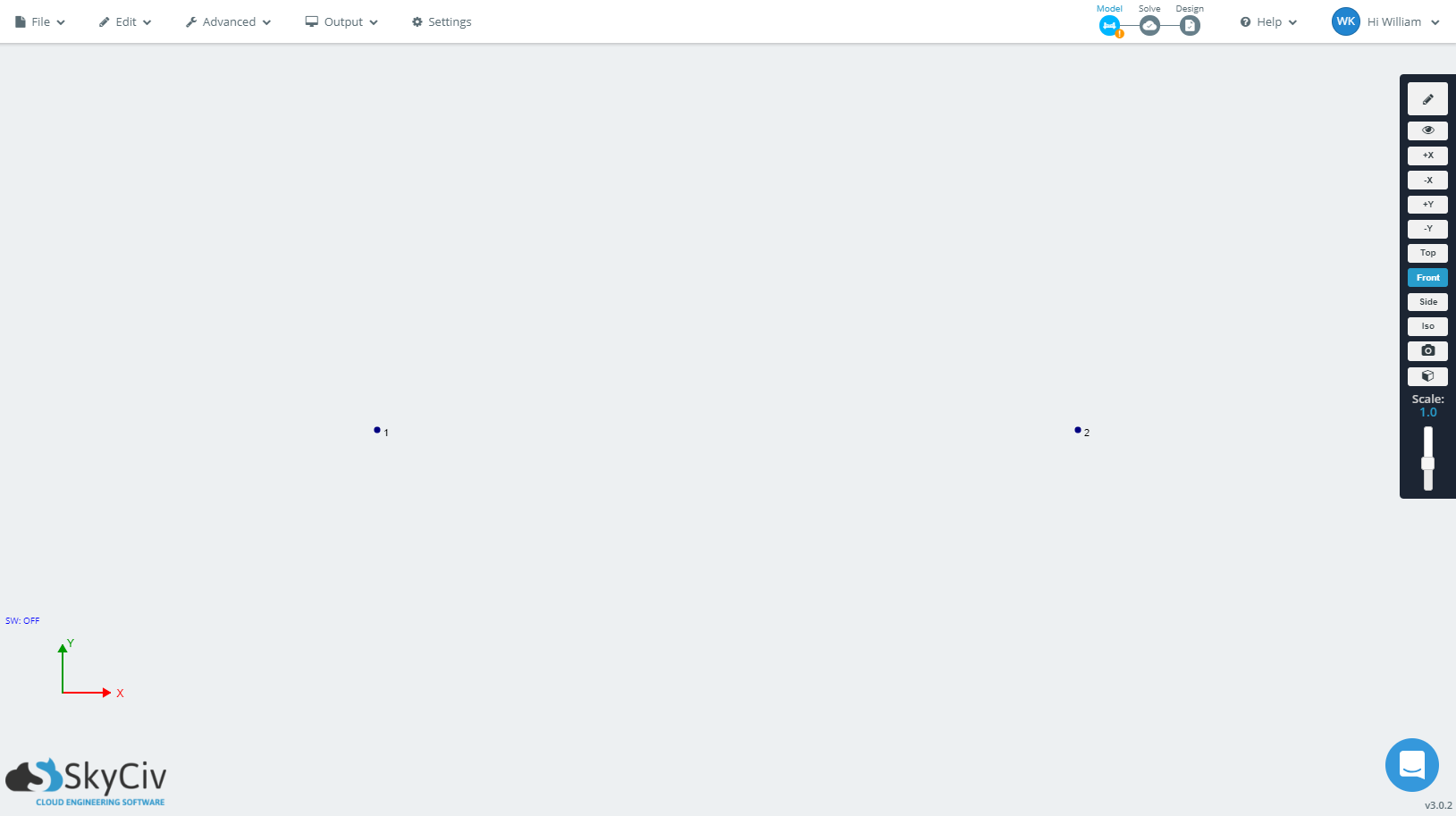Select the two nodes by holding Ctrl and clicking the two nodes. Alternatively, you can select them by holding Ctrl and pressing ‘a’ to select all. When selected, the two nodes will turn blue.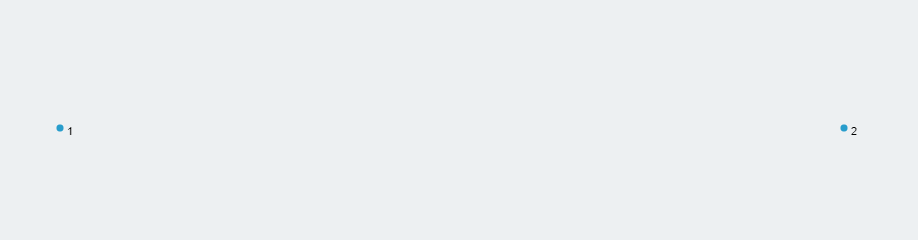Go to the top toolbar, then Edit > Operations > Curved Member.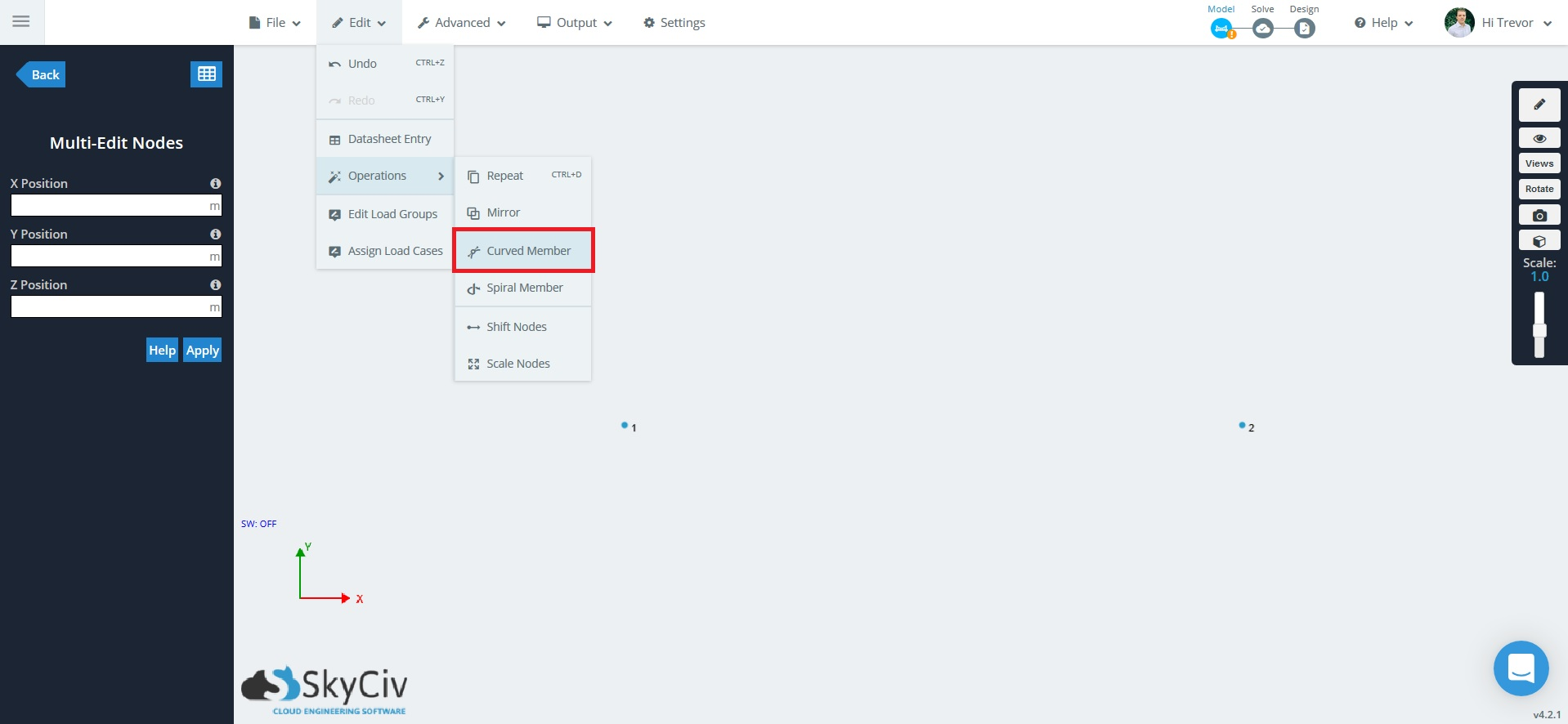A popup will appear to give you some options:

• Number of Members – The number of members used to make the curvature.
• Section ID for the Members – The cross section ID applied to each member.
• Radius – The radius of the curve between the two nodes. The minimum value of the radius is half the distance between the nodes
• Plane – Which coordinate plane the curve will be generated in.
• Direction – Positive or Negative, affects which direction the curve “travels”

For an example, we will use 20 members to make up a curve (the more members, the more accurately the curve is represented and vice versa). For the radius, the minimum value is the half distance between the two nodes. Since the two nodes in this example are separated by 10 ft, the minimum half distance is 5 ft. A value higher than the minimum for the radius will result in a ‘flatter’ curve. You can also directly specify section ID for the members of the curve to assign them a particular cross section. Plane options (XY-plane, XZ-plane) and direction (positive, negative) can also be specified.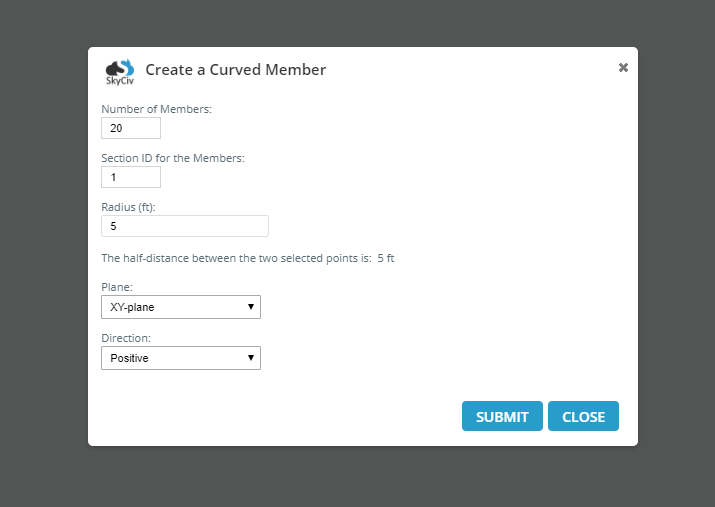The example below is used the settings as above (20 members, with radius of 5 ft).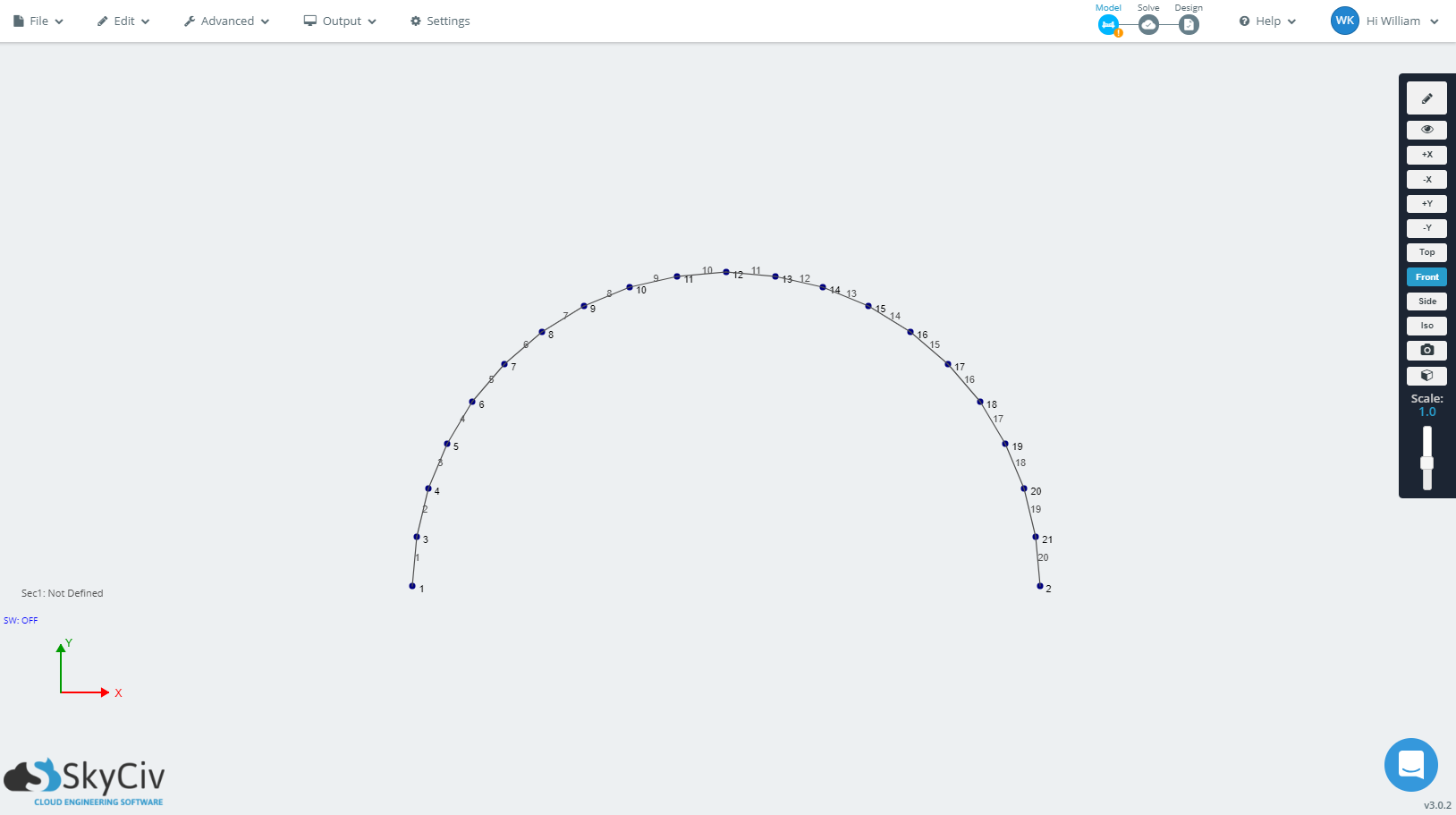You can undo the curve after you’ve generated it by holding Ctrl and pressing ‘z’. The below is an image of the above undone, and then recreated with 10 members with radius of 5 ft: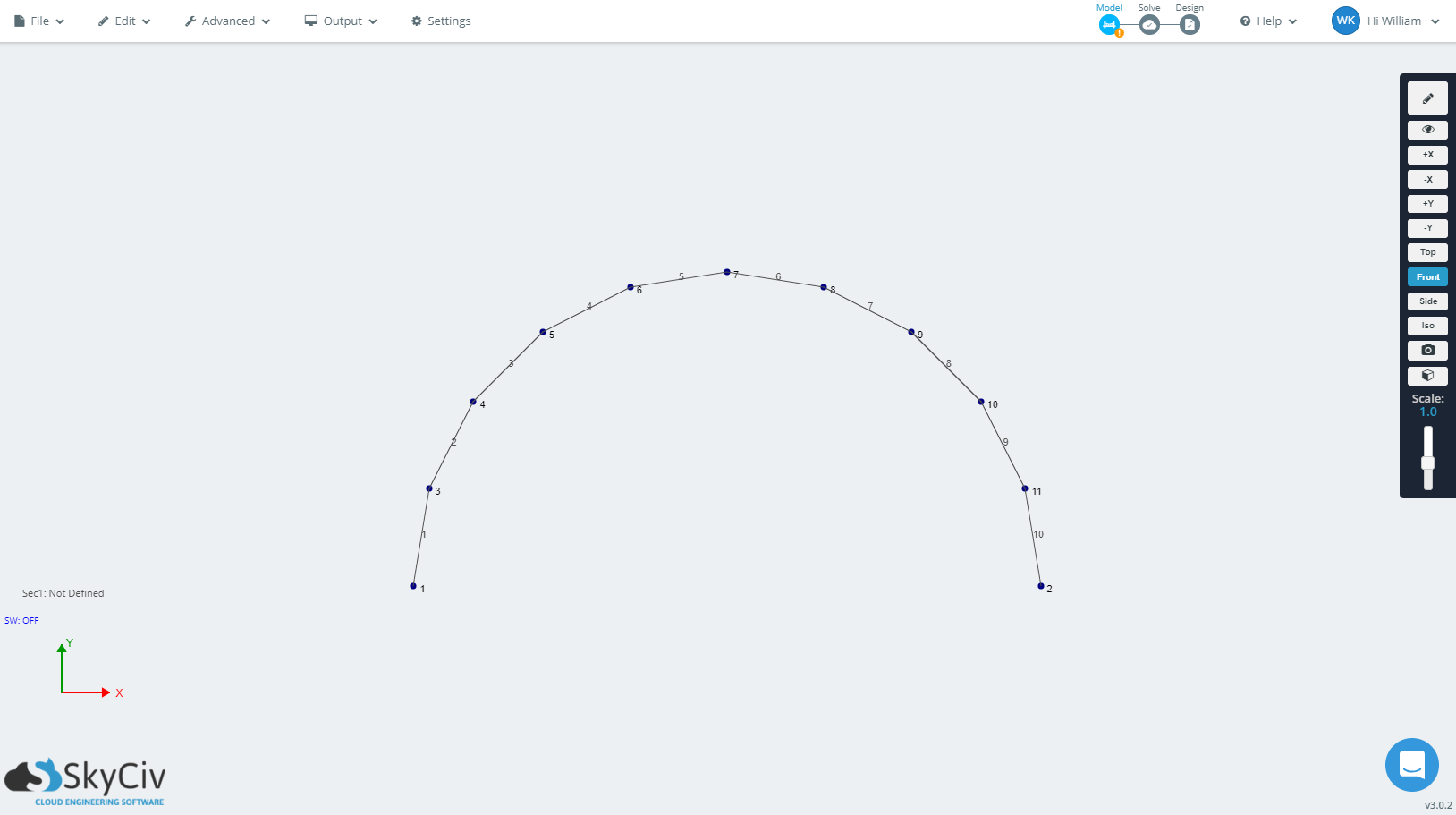The image below is of a curve with 20 members, with a radius of 8 ft.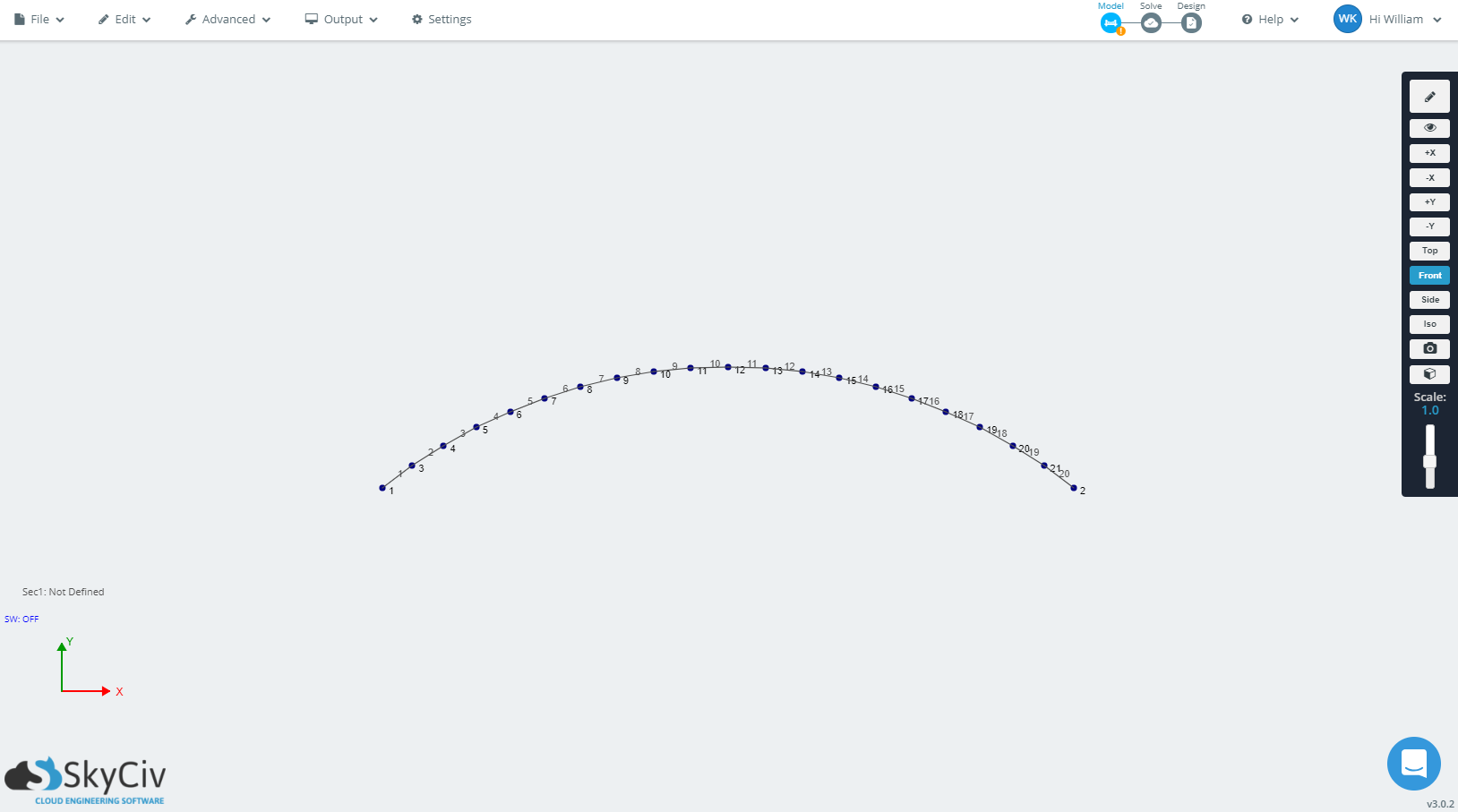# Spiral Member

The ‘Spiral Member’ operation which allows you to select one node as the starting point, and generate a spiral (made up of members). This feature is useful when modeling spiral structures like some types of staircases or railings.

## Example: Modelling a spiral structure

In this example we will model a spiral structure. The first step is to a node at the origin (0,0,0).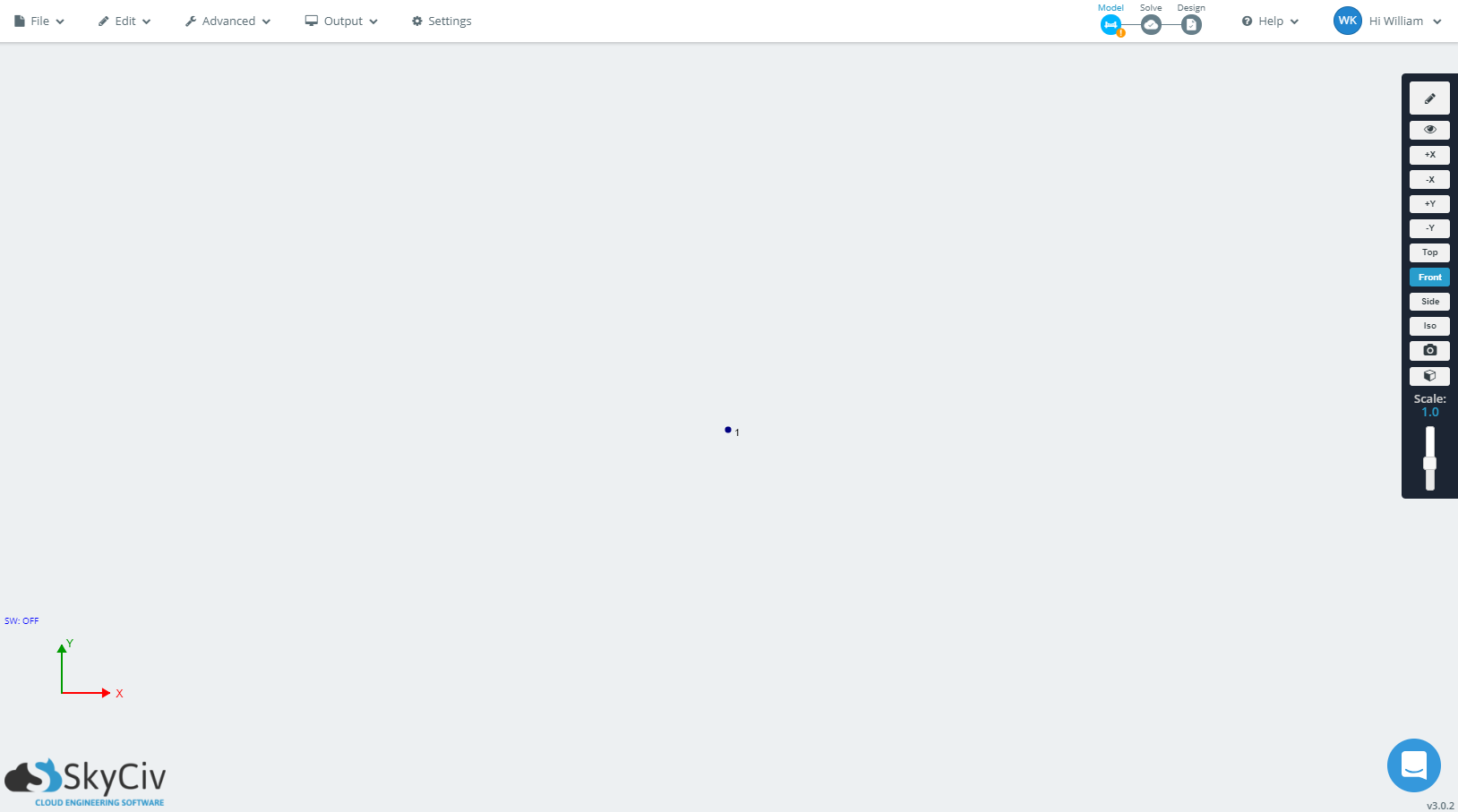Select the nodes by clicking it. When selected, the node will turn blue.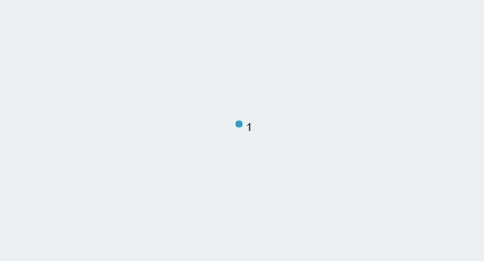Go to the top toolbar, then Edit > Operations > Spiral Member.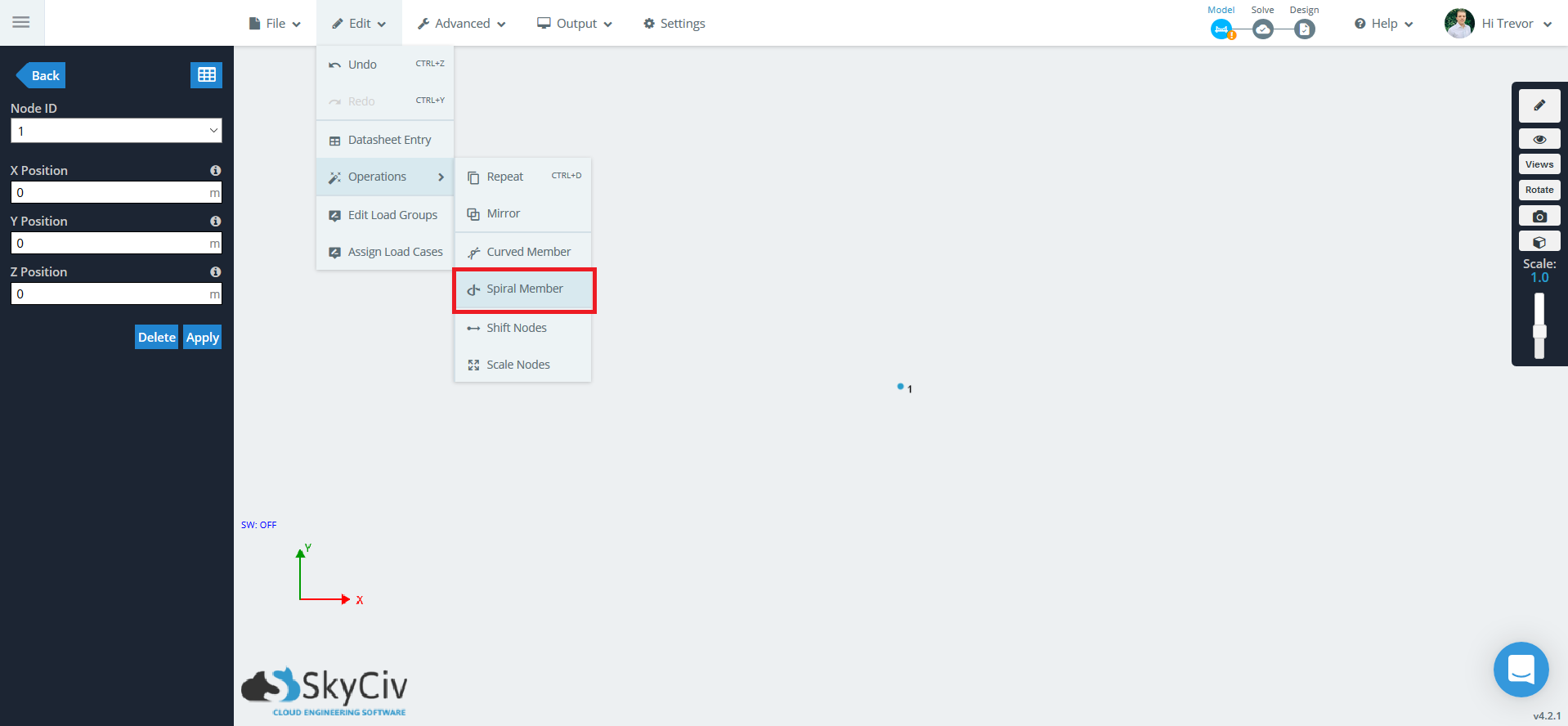A popup will appear to give you some options.

• Total Number of Members to Use – The number of members making up the spiral structure.
• Section ID for the Members – The cross section ID applied to each member.
• Helix Base Point Center Point – The center of the helix structure. Enter x, y, z coordinate.
• Helix Length – The length between the turns, in the propagation direction.
• Number of 360 degree turns – The number of complete turns.
• Direction – The spiral propagation direction.
• Turning Direction – The direction that the spiral will turn in (clockwise / counter clockwise) as it propagates in a particular direction.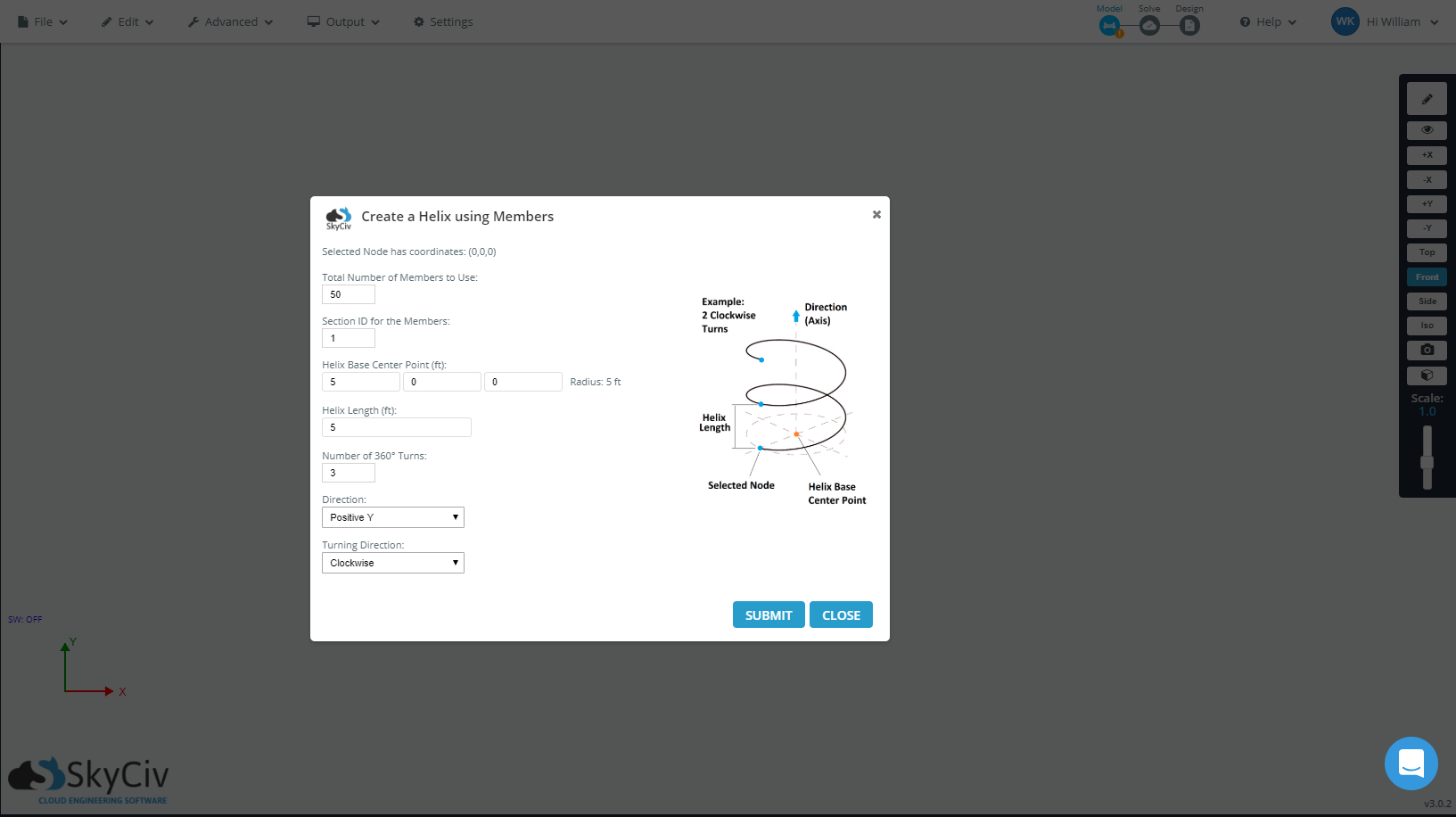The generated spiral structure is shown below.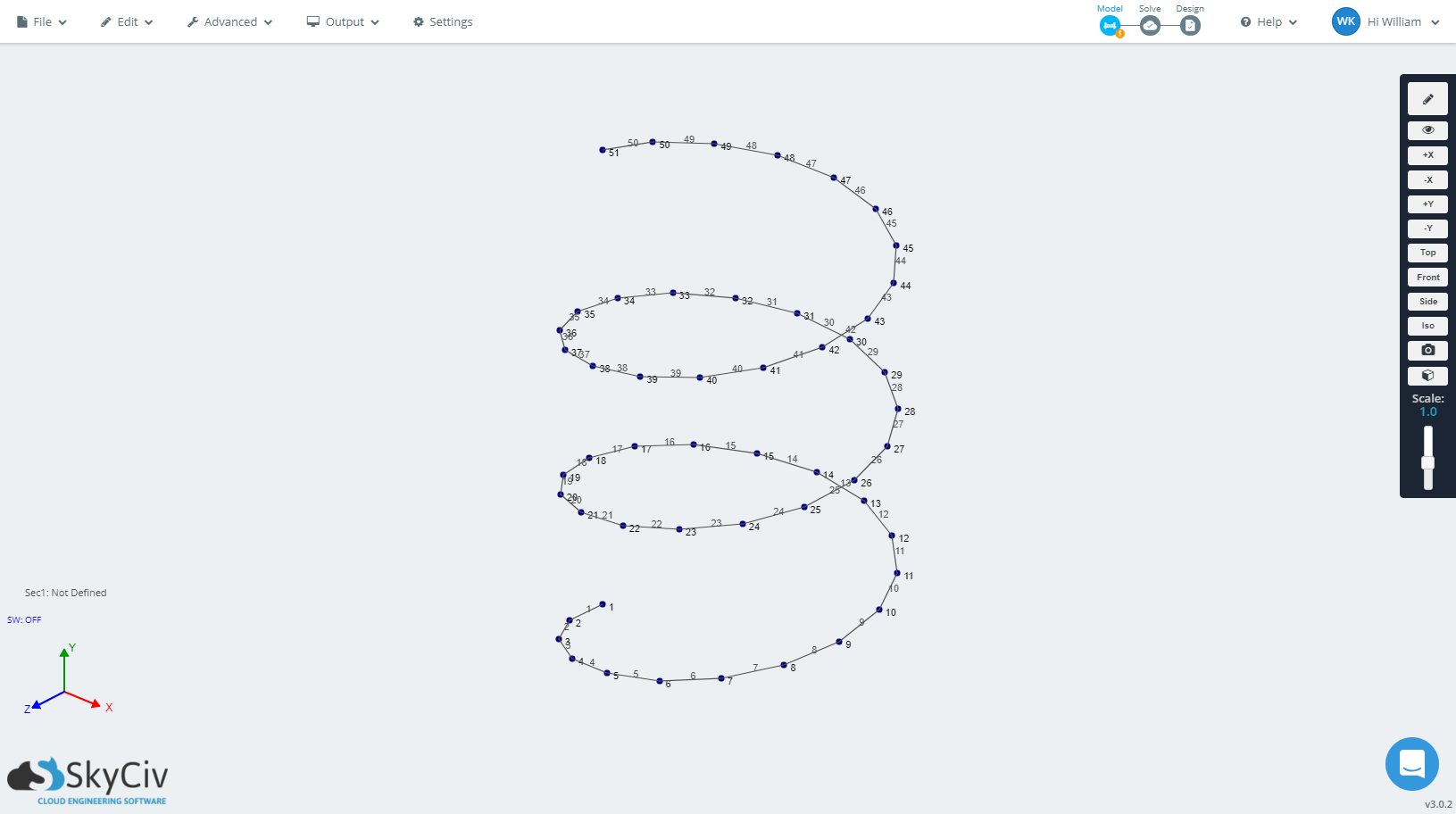test test